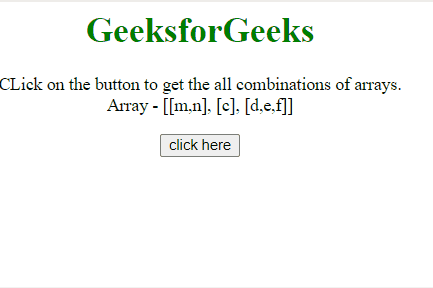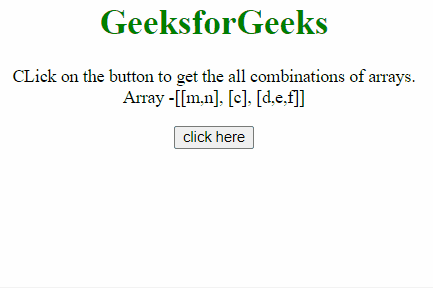Open in App
Not now

# Find all the combinations of the array values in JavaScript

• Last Updated : 19 Dec, 2022

The task is to get the cartesian product of the arrays(Finding the all combination after concatenating them). Here, 2 approaches are discussed with the help of JavaScript.

Approach 1:

• Get all arrays in an array.
• Recursion is used to solve the problem. The base condition is when the length of the array reduces to zero then return the string build till now. Else
• Reduce the first array element by .reduce() method and return the result returned from the recursion result(Recursion is called every time after leaving the first item of the array) plus the previous value in concatenation with every array element.
• Return the final ans array, which contains all combinations.

Example 1: This example implements the above approach.

## html

 `<``body` `style``=``"text-align:center;"``> ` `    ``<``h1` `style``=``"color:green;"``> ` `        ``GeeksforGeeks ` `    `` ` `    ``<``p` `id``=``"GFG_UP"``> ` `    `` ` `    ``<``button` `id``=``"button"` `onclick``=``"GFG_Fun();"``> ` `        ``click here ` `    `` ` `    ``<``p` `id``=``"GFG_DOWN"``> ` `    `` ` `    ``<``script``> ` `        ``var up = document.getElementById('GFG_UP'); ` `        ``var down = document.getElementById('GFG_DOWN'); ` `        ``var arr = [ ` `            ``['m', 'n'], ` `            ``['c'], ` `            ``['d', 'e', 'f'] ` `        ``]; ` `        ``up.innerHTML = "CLick on the button to get the "+ ` `        ``"all combinations of arrays.<``br``>Array - "+ ` `        ``"[[" + arr + "], [" + arr + "], [" + arr + "]]"; ` `         `  `        ``function getCombn(arr, pre) { ` `            ``pre = pre || ''; ` `            ``if (!arr.length) { ` `                ``return pre; ` `            ``} ` `            ``var ans = arr.reduce(function(ans, value) { ` `                ``return ans.concat(getCombn(arr.slice(1), pre + value)); ` `            ``}, []); ` `            ``return ans; ` `        ``} ` `         `  `        ``function GFG_Fun() { ` `            ``down.innerHTML = getCombn(arr); ` `        ``} ` `    `` ` ``

Output:Approach 2:

• Get all arrays in an array.
• Recursion is used to solve the problem. The base condition is When the length of the array reduces to one then return that element of the array. Else
• Call recursion after leaving the first element of the array and store the result in a variable(otherCases).
• Loop through every element of the Array(otherCases) and inside every element loop through the first element of the Array(arr).
• Concatenate every element of the Array(arr) with the Array(otherCases) and push the result in the answer array.

Example 2: This example implements the above approach.

## html

 `<``body` `style``=``"text-align:center;"``> ` `    ``<``h1` `style``=``"color:green;"``> ` `        ``GeeksforGeeks ` `    `` ` `    ``<``p` `id``=``"GFG_UP"``> ` `    `` ` `    ``<``button` `id``=``"button"` `onclick``=``"GFG_Fun();"``> ` `        ``click here ` `    `` ` `    ``<``p` `id``=``"GFG_DOWN"``> ` `    `` ` `    ``<``script``> ` `        ``var up = document.getElementById('GFG_UP'); ` `        ``var down = document.getElementById('GFG_DOWN'); ` `        ``var arr = [ ` `            ``['m', 'n'], ` `            ``['c'], ` `            ``['d', 'e', 'f'] ` `        ``]; ` `        ``up.innerHTML = "CLick on the button to get the all "+ ` `        ``"combinations of arrays.<``br``>Array -"+ ` `        ``"[[" + arr + "], [" + arr + "], [" + arr + "]]"; ` `         `  `        ``function getCombn(arr) { ` `            ``if (arr.length == 1) { ` `                ``return arr; ` `            ``} else { ` `                ``var ans = []; ` `                 `  `                ``// recur with the rest of the array. ` `                ``var otherCases = getCombn(arr.slice(1)); ` `                ``for (var i = 0; i < ``otherCases.length``; i++) { ` `                    ``for (var ``j` `= ``0``; j < arr.length; j++) { ` `                        ``ans.push(arr[j] + otherCases[i]); ` `                    ``} ` `                ``} ` `                ``return ans; ` `            ``} ` `        ``} ` `         `  `        ``function GFG_Fun() { ` `            ``down.innerHTML` `= ``getCombn``(arr); ` `        ``} ` `    `` ` ``

Output:My Personal Notes arrow_drop_up
Related Articles